Question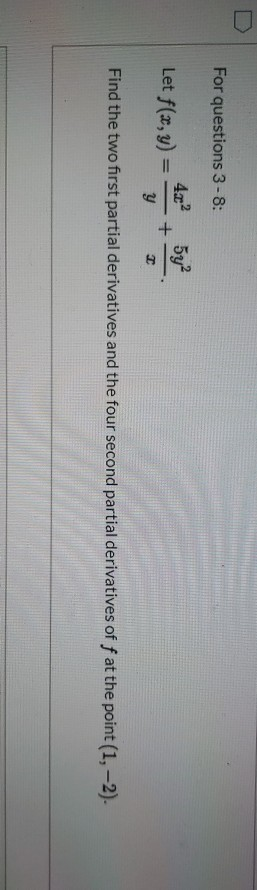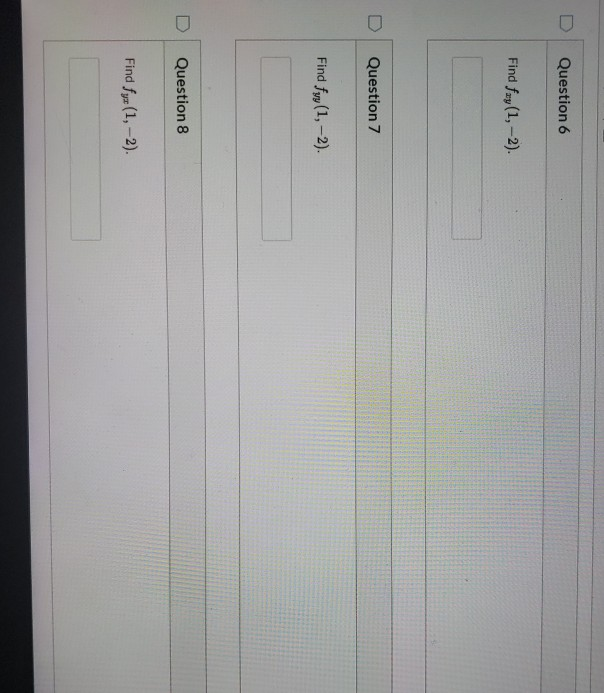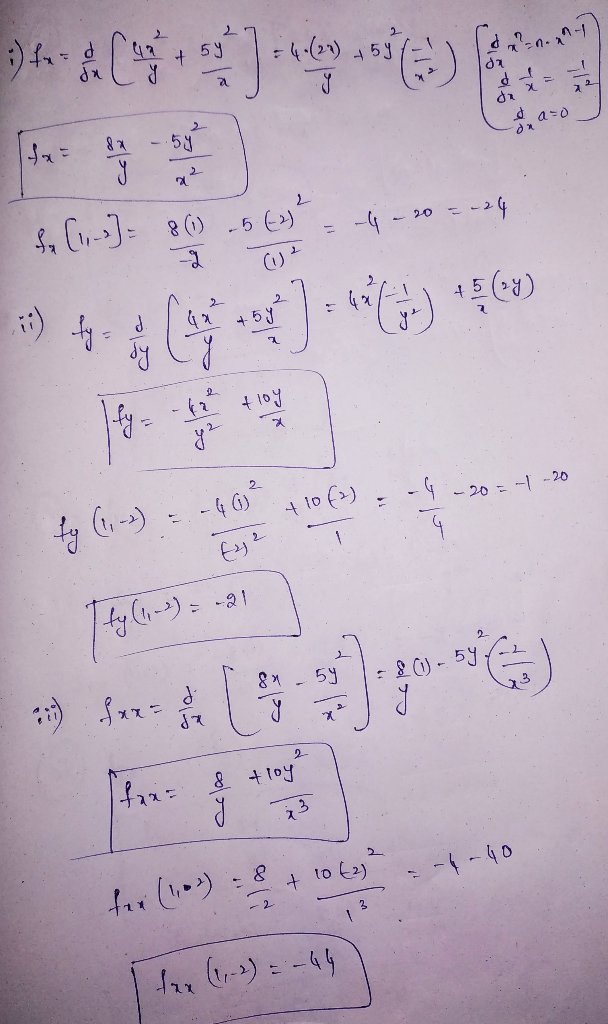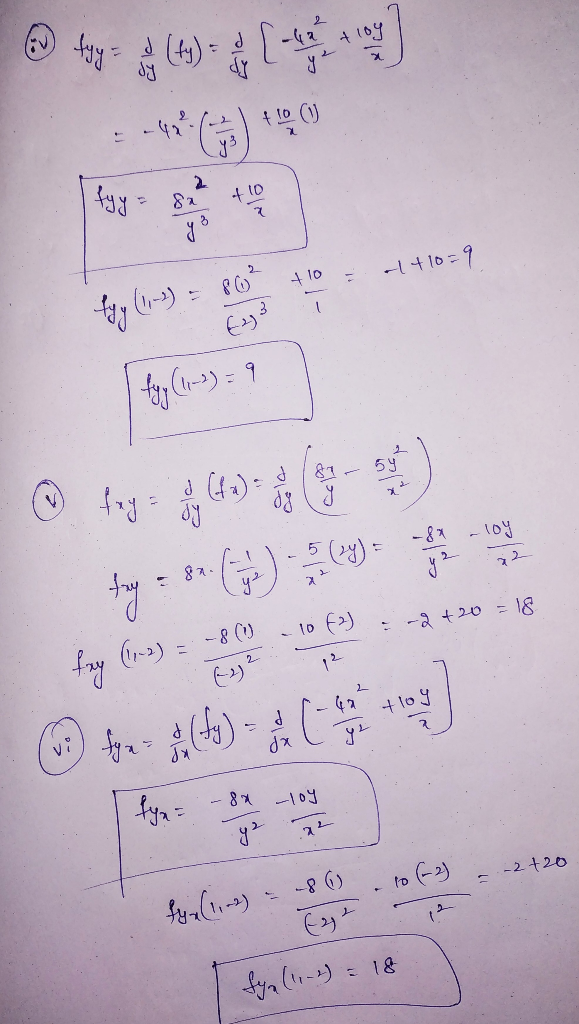#### Earn Coins

Coins can be redeemed for fabulous gifts.

Similar Homework Help Questions
• ### Find all the first and second order. partial derivatives of f(x, y) = 8 sin(2x +...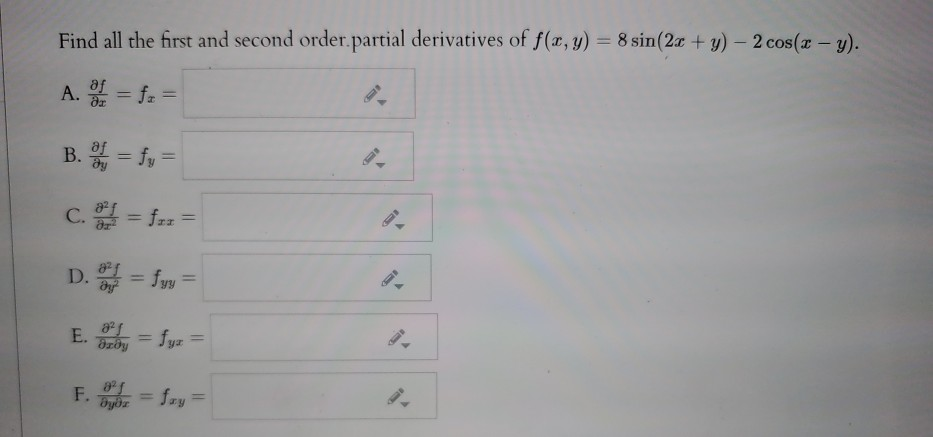Find all the first and second order. partial derivatives of f(x, y) = 8 sin(2x + y) - 2 cos(x - y). A. SI = fr = B. = fy = c. = f-z = D. = fyy = E. By = fyz = F. = Sxy=

• ### Find the first partial derivatives of the function. f(x, y) = 2x + 4y + 8...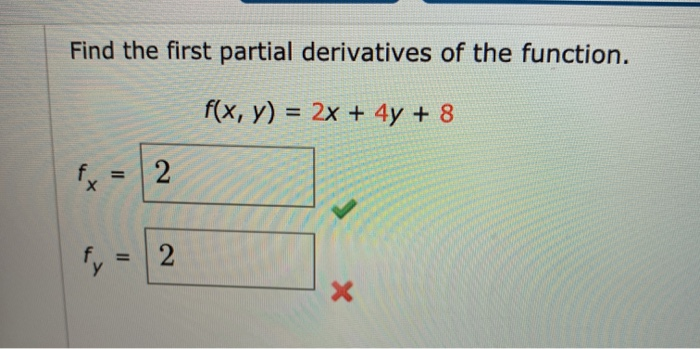Find the first partial derivatives of the function. f(x, y) = 2x + 4y + 8 fy 2 fy = 2 X

• ### Problem 8. (1 point) (1 pt) Calculate all four second-order partial derivatives of f(x,y) = 2x²y...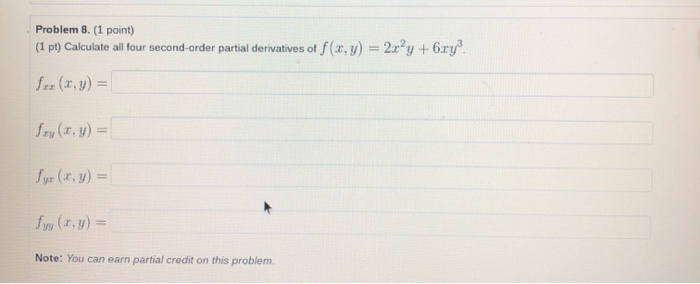Problem 8. (1 point) (1 pt) Calculate all four second-order partial derivatives of f(x,y) = 2x²y +6.ry? for (x,y) = Szy(x, y) = Syr (x, y) = fry (x. y) = Note: You can earn partial credit on this problem.

• ### (10 points) Find all first and second partial derivatives of f(x,y) = 24 – 3.z”y2 +...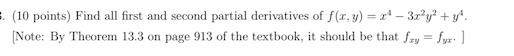(10 points) Find all first and second partial derivatives of f(x,y) = 24 – 3.z”y2 + y* [Note: By Theorem 13.3 on page 913 of the textbook, it should be that fry = fyr:]

• ### Problem 5. (1 point) Find all the first and second order partial derivatives of f(x,y) 7...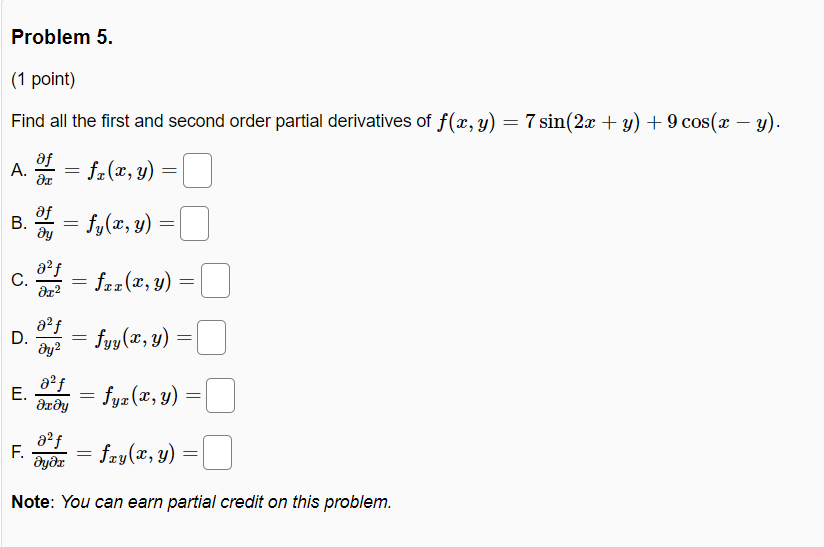Problem 5. (1 point) Find all the first and second order partial derivatives of f(x,y) 7 sin(2x + y) + 9 cos(x - y). A. = fx(x,y) = B. = fy(x, y) = af C. ar2 = fcz(x, y) = af D. ay2 = fyy(x,y) = E. af деду fyz(x, y) = af F. მყმz = fxy(x, y) = Note: You can earn partial credit on this problem.

• ### Problem #8: Let f(x, y, z) = xzly. Find the value of the following partial derivatives....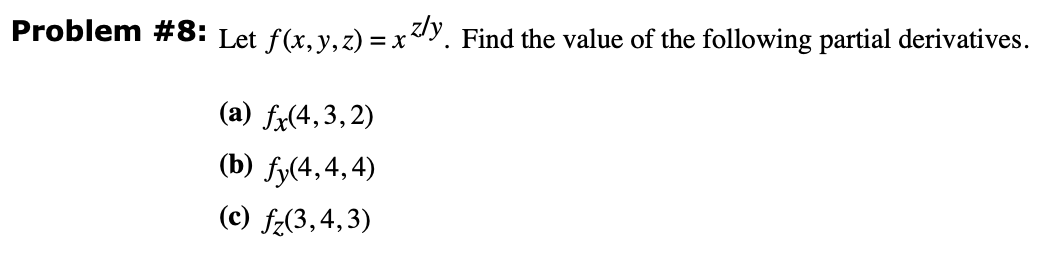Problem #8: Let f(x, y, z) = xzly. Find the value of the following partial derivatives. (a) fx(4,3,2) (b) fy(4,4,4) (c) fz(3,4,3)

• ### find all first partial derivatives f(x,y)= 5x^3+4y-3 Find all first partial derivatives. f(x, y) = 5x3...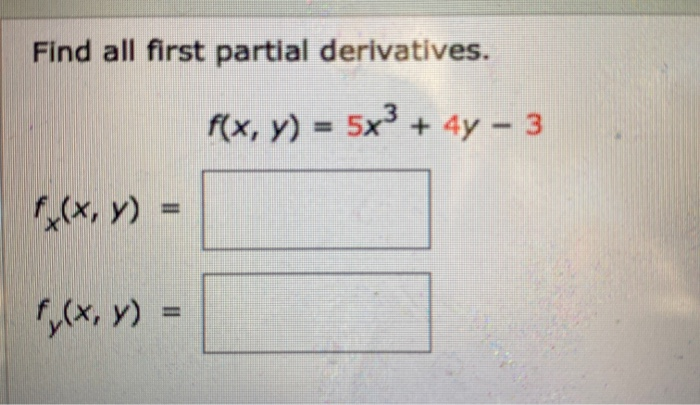find all first partial derivatives f(x,y)= 5x^3+4y-3 Find all first partial derivatives. f(x, y) = 5x3 + 4y - 3 f(x,y) = f(x,y) =

• ### ** Please answer two parts of the questions. Part 2 out of 2: f y (1,0)...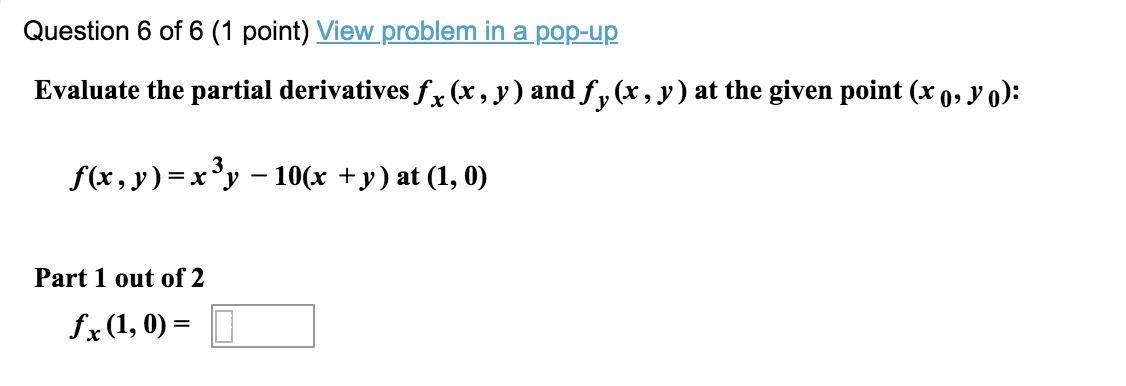** Please answer two parts of the questions. Part 2 out of 2: f y (1,0) = _________________ Question 6 of 6 (1 point) View problem in a pop-up Evaluate the partial derivatives fx (x, y) and fy(x, y) at the given point (x 0, yo): f(x, y)= x3y – 10(x + y) at (1, 0) Part 1 out of 2 fx (1,0) =

• ### Let f(x, y, z)=zxly. Find the value of the following partial derivatives. (a) f(4,4,3) (b) fy(4,...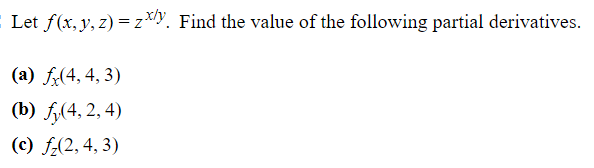Let f(x, y, z)=zxly. Find the value of the following partial derivatives. (a) f(4,4,3) (b) fy(4, 2, 4) (c) f(2,4,3)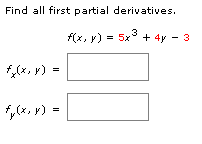Please help me answer these 2 questions Find all first partial derivatives. f(x, y) = 5x + 4y - 3 (, y) = f(x, y) = = Differentiate implicitly to find dy dx xx2 x + y = 5 dy II